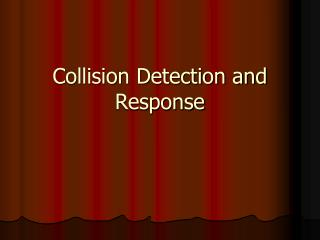DownloadDownload PresentationCollision Detection and Response

# Collision Detection and Response

Download Presentation## Collision Detection and Response

- - - - - - - - - - - - - - - - - - - - - - - - - - - E N D - - - - - - - - - - - - - - - - - - - - - - - - - - -
##### Presentation Transcript

1. Collision Detection and Response

2. Motivation • OpenGL is a rendering system • OpenGL has no underlying knowledge of the objects it draws • Collision Detection == Intersection Detection

3. Motivation • Collision Detection • Identifying the intersection of 3D models • Collision Response • Calculating the appropriate post collision response of the 3D models • Assumptions • Closed 3D polygonal objects

4. Overall Approach • Where is collision detection and response in code? • In animation function • Usually in Idle or Display • Update object positions • If (CollisionDetection()) • Collision Response() • Draw Objects

5. Collision Detection • Basic Collision Detection function • for (i=0;i<num_of_objects-1;i++) • for (j=i+1;j<num_of_objects;j++) • X=TestIntersection(Object I, Object j) • If (x==1) return 1;

6. Collision Detection Packages • SWIFT++ • http://www.cs.unc.edu/~geom/SWIFT++/ • Works on ‘polygon soup’

7. Why is collision detection difficult? • Too many calculations • Reduce # of calculations • Accuracy • Accept approximations • Lots of poor cases • Guarantee detection? • Accuracy requirements? • http://www.cs.unc.edu/~geom/collide/index.shtml

8. Reduce Calculations • Reduce object complexity • Test with only other objects that might be within collision spaces • Spatial divisions • Pre-process vs. runtime

9. Approximate Object Shape • For many applications, it is not necessary to get absolute collision detection accuracy • How can we approximate the shape?

10. Bounding Boxes • Calculate a 3D box that bounds the object • In the collision detection test, use the 3D box to test for collision in place of the full model • Two types • Axis Aligned • Bounding boxes are aligned w/ the world coordinate axes • Object Aligned • Bounding boxes are aligned w/ the object coordinate axes

11. Axis Aligned Bounding Boxes • How do we calculate a AABB? • Take max and min for each dimension • Pros • Very fast • Works for many situations • Cons • Collision detection accuracy reduced for many cases

12. Axis Aligned Bounding Boxes • How do we calculate intersections? • Calculate overlap for the min-max range for each dimension • Pros • Very fast • Works for many situations • Cons • Collision detection accuracy reduced for many cases

13. Object-Aligned Bounding Boxes • Calculate BB based on object’s primary dimensions • Object’s dimensions could be encoded in model or calculated real-time • Apply M matrix to bounding box coordinates

14. Other bounding shapes • Boxes • Cubes • Cylinders • Polygons • User-defined shapes • Should be easy to calculate and test

15. Bounding Box Approach • Test collisions with BB • Can either report collision • Or then calculate more accurate collision • Benefits of this include more accurate response

16. Poor cases • Thin objects

17. Poor cases • Numerical Precision

18. Collision Response • Assuming rigid bodies • Idea is to “rewind time” and to point of collision and calculate resulting location

19. Why is collision response difficult? • Resolving interpenetration is difficult • Multiple objects http://www.peroxide.dk/download/tutorials/tut10/pics/pic04.jpg

20. Numerical Imprecision • Vibrations • Solutions? Minimum delta